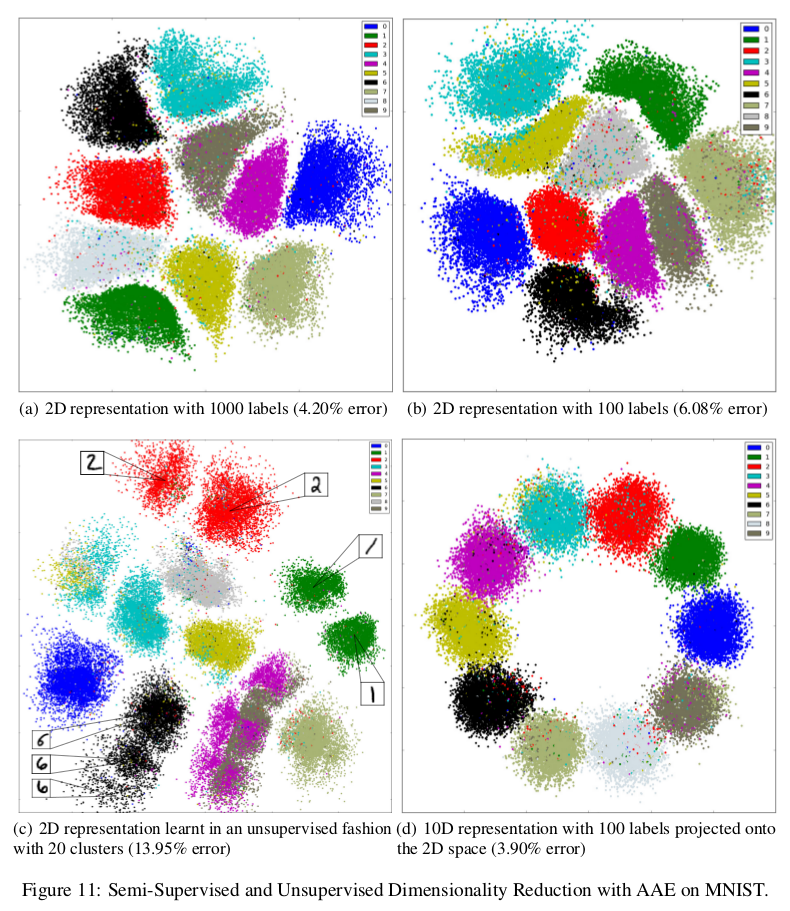## Summary

The Adversarial Autoencoder (AAE) is a probabilistic autoencoder that uses GANs to match the posterior of the hidden code with an arbitrary prior distribution. This way, sampling from the prior space produces meaningful samples. As a result, the decoder learns a mapping from the imposed prior to the data distribution.

## Model

### Basic architecture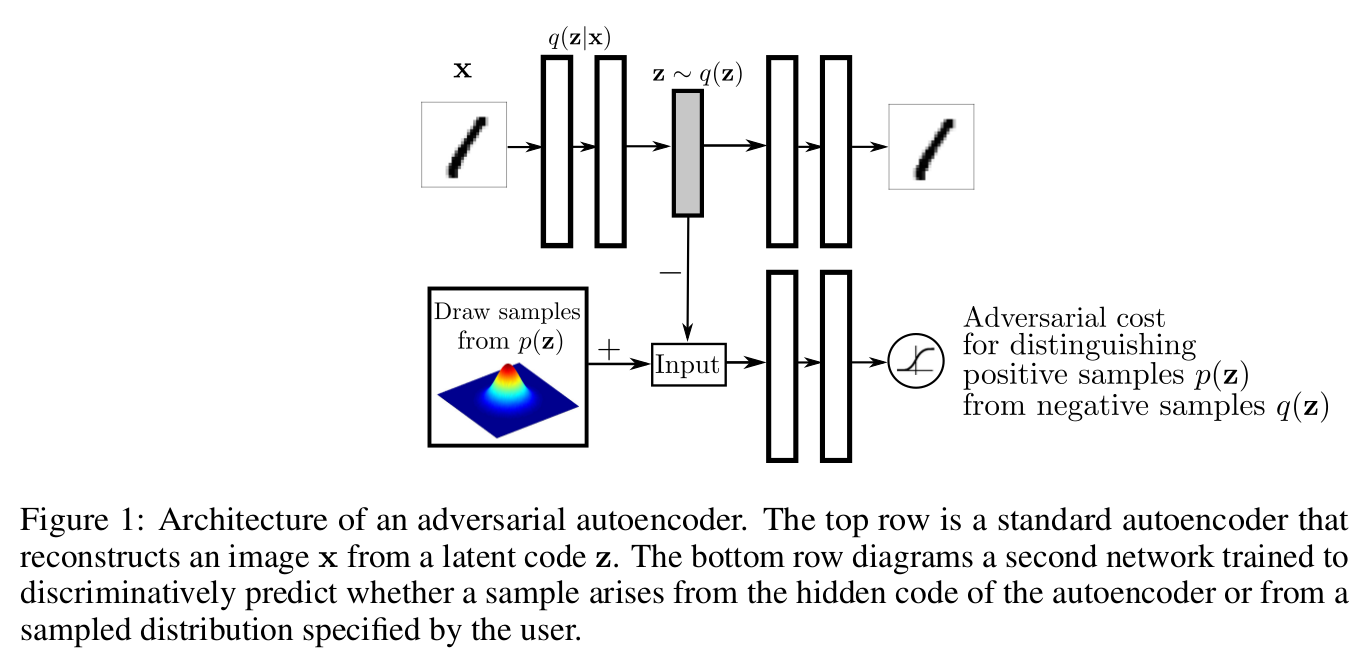Note that the top row is a standard variational autoencoder, and the bottom row is a discriminator trying to identify samples from the hidden code vs samples from the user-chosen prior.

### Choices of encoder

• The encoder can be deterministic; in this case, the model is a standard autoencoder.

• It can also define a gaussian posterior like variational autoencoders; in this case, the encoder outputs the mean and variance of the gaussian conditioned on the input sample. The reparametrization trick can be used to back-propagate through the encoder.

• Finally, the encoder can be a universal approximation of the posterior. It takes as input a sample $$\mathbf x$$ and random gaussian noise $$\eta$$.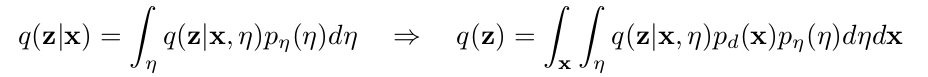In theory, the universal approximator is more powerful but still easy to train, but the authors mention that the results were all similar no matter what kind of encoder was used, so all reported results are from the deterministic encoder…

### Difference with Variational Autoencoders

Variational autoencoders use a KL-Divergence loss to optimize a lower bound and impose a prior on the hidden code, while the AAE uses adversarial training to match the posterior to the prior.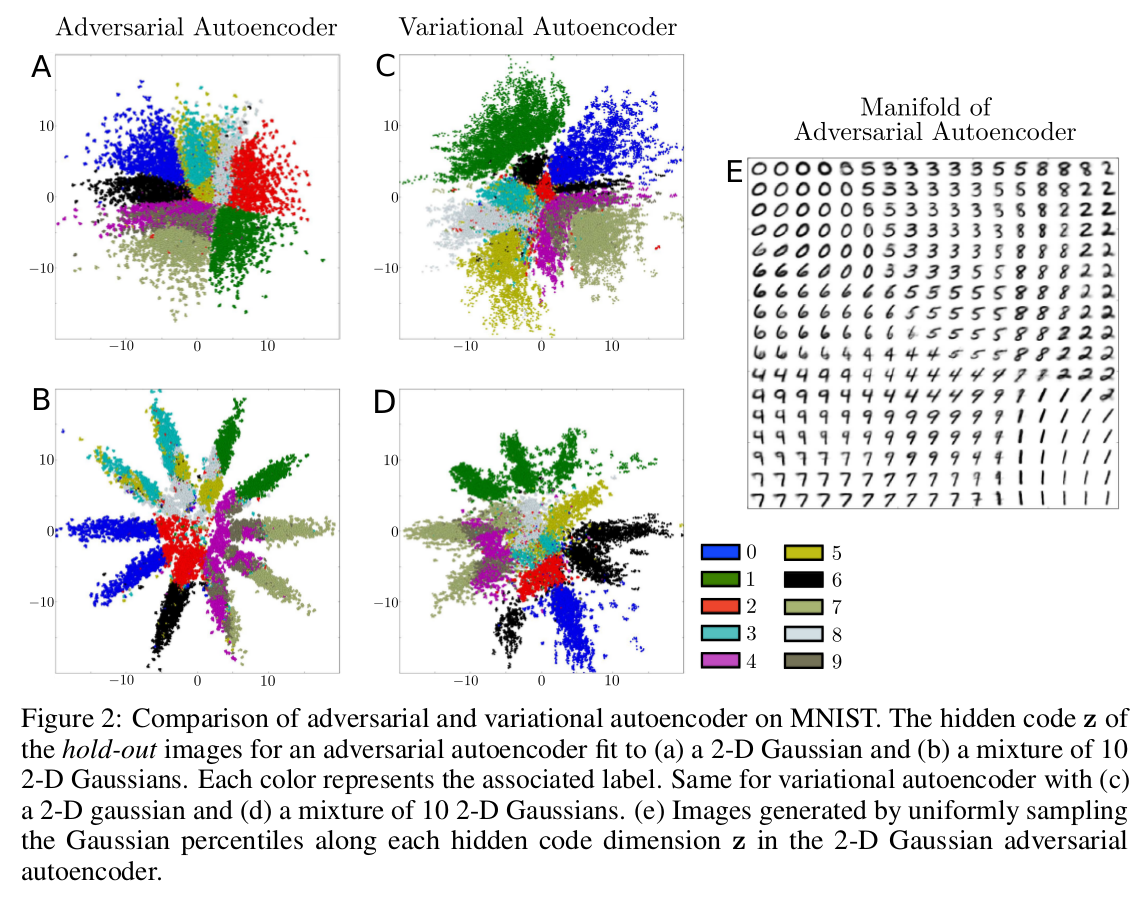A one-hot class vector is added as input to the discriminator, and includes an extra class for unlabeled examples.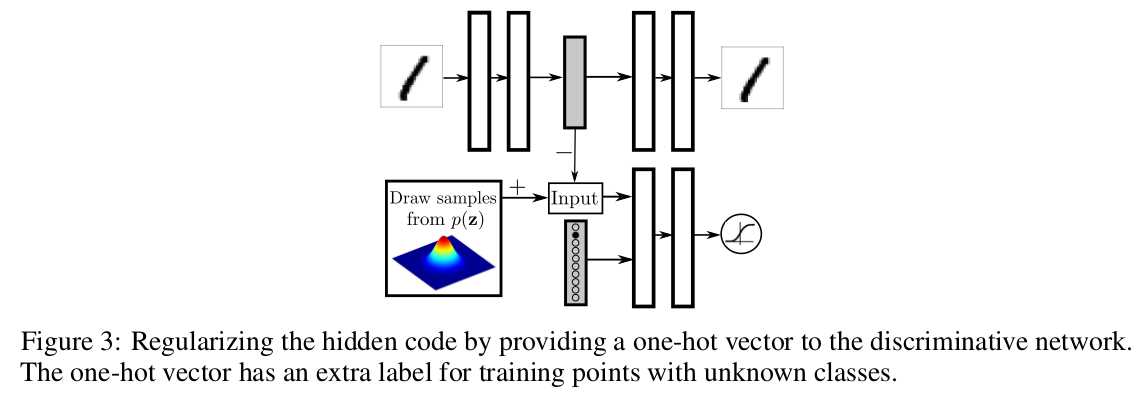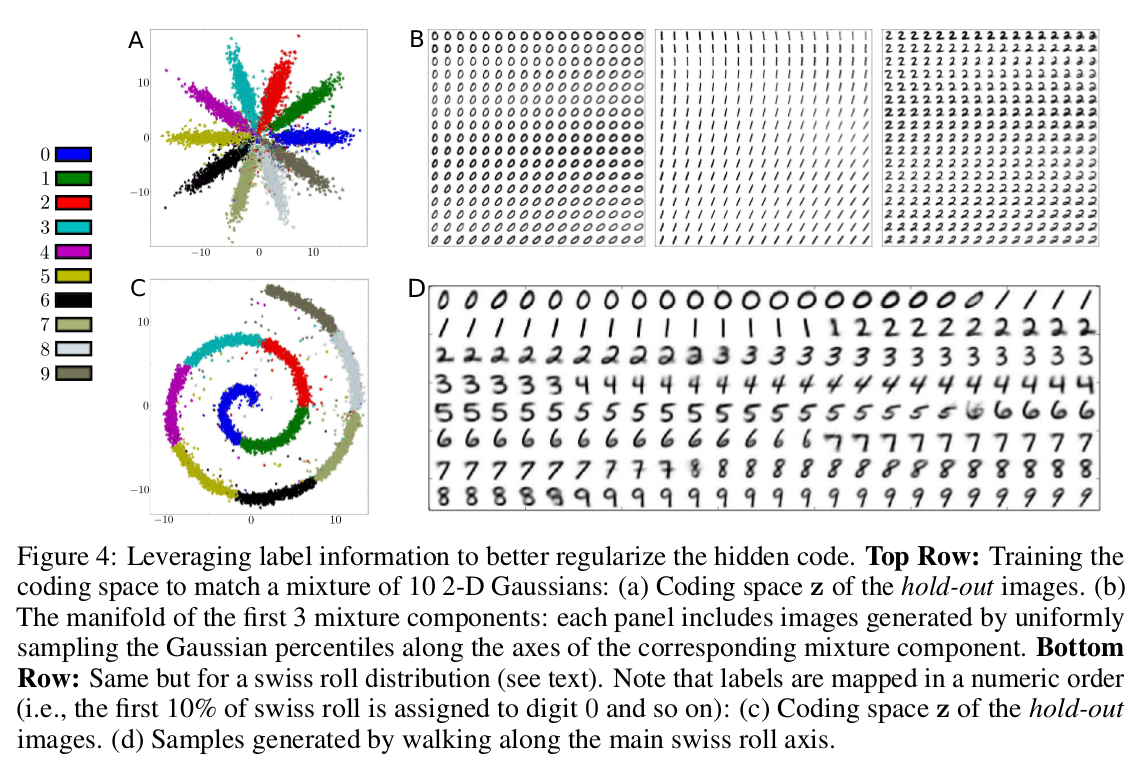## Supervised AAE

The class label is given to the decoder, which means the hidden representation only needs to encode the “style”.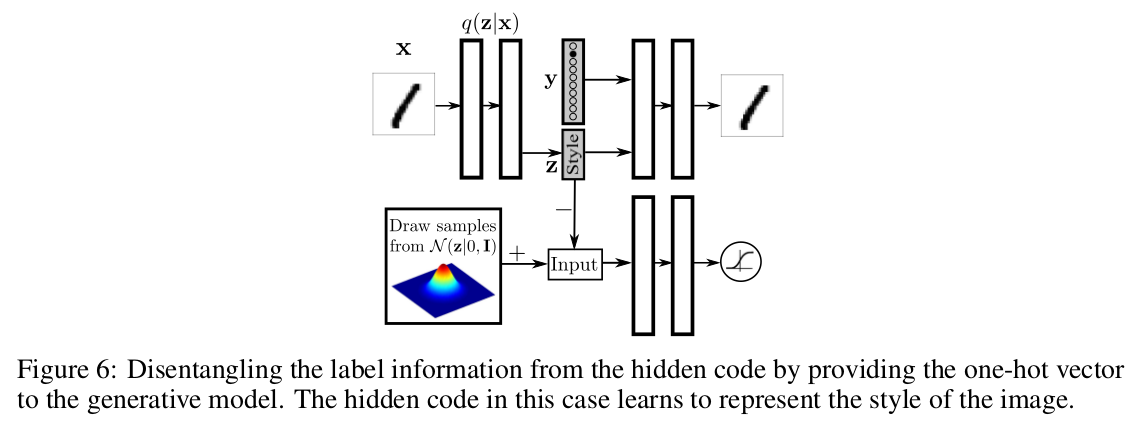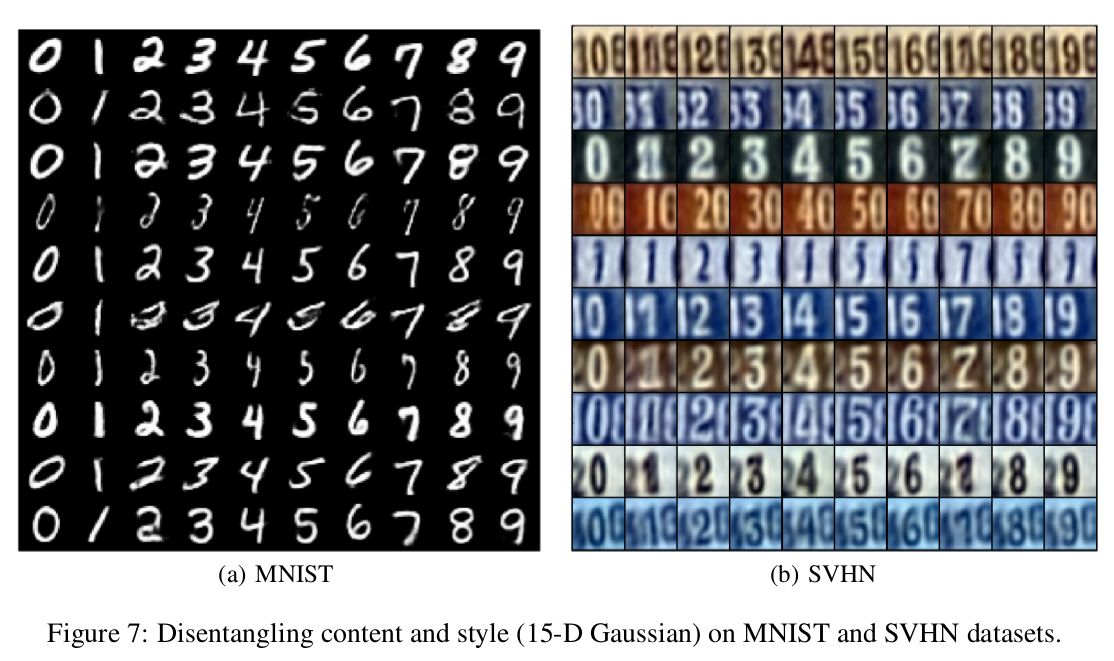In Figure 7, a hidden code is sampled for each row, and only the label changes.

## Semi-Supervised AAE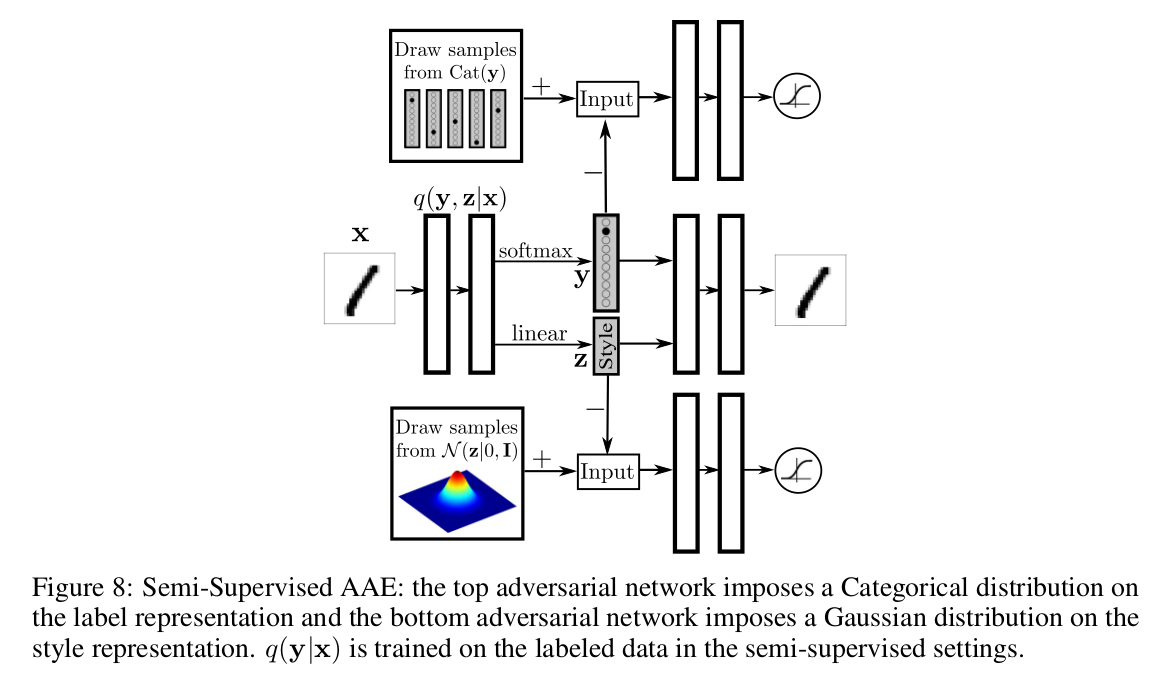Training is done in 3 phases:

• Reconstruction phase: Update the autoencoder to minimize the reconstruction error.
• Regularization phase: Update both discriminative networks, then update the generator to fool them.
• Semi-Supervised Classification phase: Update the encoder to minimize the cross-entropy on a labeled batch.

Results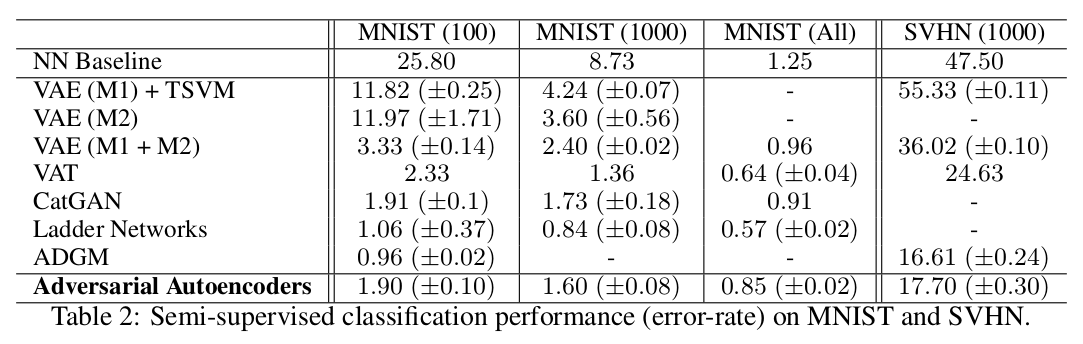AAE does better than the Variational Autoencoder, but is beaten by the Ladder Networks and ADGM.

## Unsupervised clustering

The model is the same as in Figure 8, but the number of classes now represents the number of desired clusters, and there is no classification training phase. In Figure 9, the cluster head is chosen by setting the style hidden code to zero and setting the label variable to one of the possible one-hot vectors (16 in this case).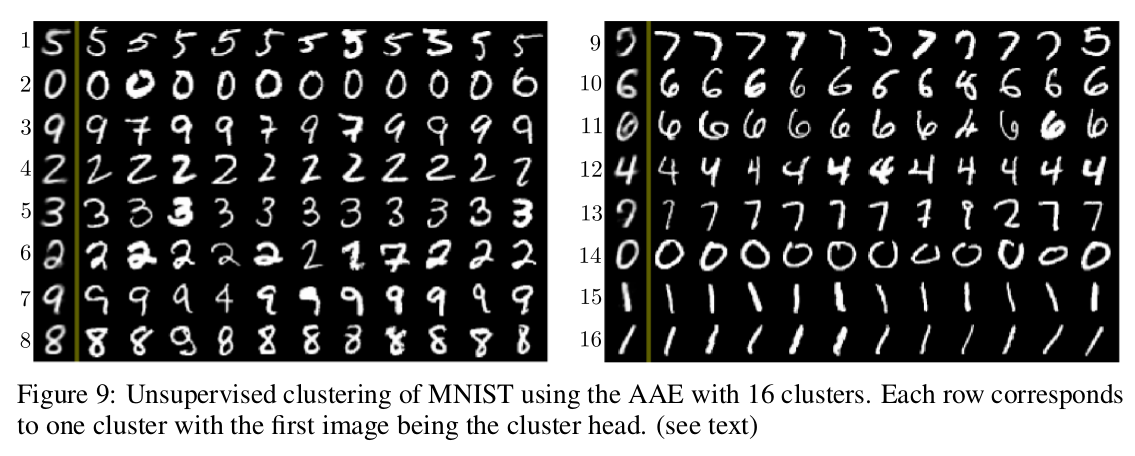## Dimensionality reduction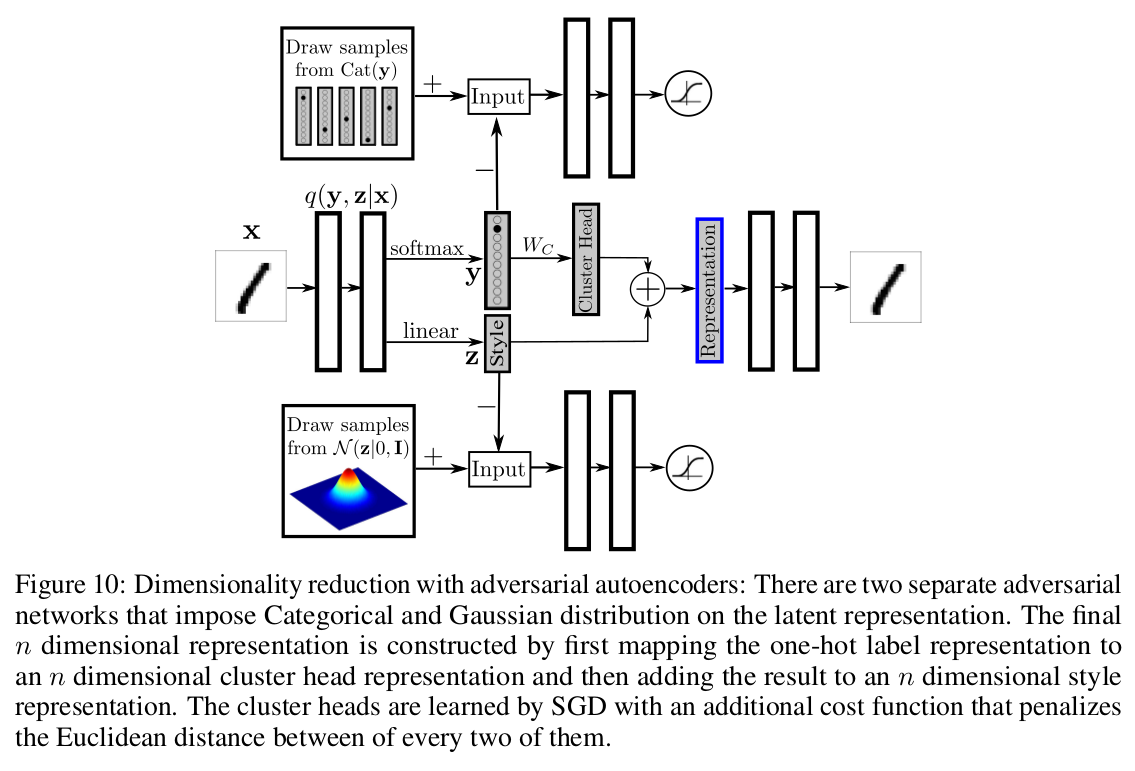The cluster head representation is obtained by multiplying the $$m$$ dimensional one-hot class label vector by an $$m × n$$ matrix $$W_C$$, where the rows of $$W_C$$ represent the $$m$$ cluster head representations that are learned with SGD ($$n$$ is the desired dimensionality).